###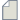Author Topic: Query regarding calculation of capacitance using ATK  (Read 512 times)

0 Members and 1 Guest are viewing this topic.

####harkishan_dua

• Regular ATK user
•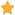• Posts: 15
• Country: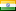• Reputation: 0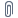##### Query regarding calculation of capacitance using ATK
« on: December 30, 2020, 16:49 »
I have a graphene bilayer structure whose capacitance I wish to find out using QuantumATK. I have made it into a device configuration with 6 layers and L-R electrodes I have followed the steps as given in the tutorial https://docs.quantumatk.com/tutorials/atomic-scale_capacitance/atomic-scale_capacitance.html for the calculation of atomic scale capacitance. And I am facing some problems and some queries that I wished to clarify.
1.   When the capacitance is being calculated using the induced charge method (Mulliken charge), I am getting a very high value of the plate distance d. When viewed from the structure, the actual  value of plate distance d = 10.26 Angstrom whereas from the induced charge analysis, I am getting the value of plate distance as 160.84 Angstrom. I am aware that the value of distance is calculated from the capacitance value. But even then why am I getting such a value of capacitance?  Attached along with are theinput .py file along with log file (mulliken_analysis.py and mulliken_analysis.log) and the induced charge plot (Device_Graphene_mulliken.png).
2.   When I am calculating the value of capacitance using the electrostatic energy analysis, I am getting a plot of Induced density at 1 volt (Device_Graphene_induced.png file) which has two maximas (dips). I wanted to know whether the distance between the dips is same as that of the plate distance d?

####Petr Khomyakov

• QuantumATK Staff
• Supreme ATK Wizard
•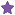• Posts: 1275
• Country:• Reputation: 24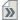##### Re: Query regarding calculation of capacitance using ATK
« Reply #1 on: January 8, 2021, 11:31 »
Could you clarify what capacitance you are trying to compute? You mentioned capacitance of bilayer graphene. Would you then mean quantum capacitance as discussed in-here https://en.wikipedia.org/wiki/Quantum_capacitance?  Otherwise, you are now calculating capacitance of a capacitor comprised of graphite electrodes for a given separation distance between the graphite plate. In that case I am sure that you have enough monolayers for your plates.

####harkishan_dua

• Regular ATK user
•• Posts: 15
• Country:• Reputation: 0##### Re: Query regarding calculation of capacitance using ATK
« Reply #2 on: January 9, 2021, 12:13 »
I am not trying to calculate the quantum capacitance. I just wanted the capacitance for the structure that is dependent on the interlayer distance between the bilayer system. But I am unable to perform calculations on a bilayer structure as when I transform it into a device configuration, more layers are added by the VNL. what should I do?

####Petr Khomyakov

• QuantumATK Staff
• Supreme ATK Wizard
•• Posts: 1275
• Country:• Reputation: 24##### Re: Query regarding calculation of capacitance using ATK
« Reply #3 on: January 12, 2021, 14:02 »
Device calculation means that you have a central region of whatever system sandwiched between two semi-infinite electrodes, this is why these layers are added, in fact, the represent semi-infinite graphite-like leads.